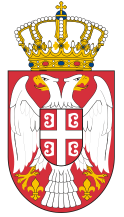Dokument se učitava

# Signal ProcessingCourse code: 317111 | 8 ECTS credits

Basic information
Level of Studies: Master applied studies
Year of Study: 1
Semester: 2
Requirements:
Goal: Signal processing introduction. Principles of signal processing and practical applications.
Outcome: Students can understand the principles and capabilities of signal processing and its visualization through practical examples and application possibilities.
Contents of the course
Theoretical instruction:
1. Introduction.
2. Signal processing history. Application examples.
3. Signal visualization (Matlab, Python, Excel).
4. Discrete complex exponential signal. Music signal synthesis.
5. Fourier analysis. Discrete-time Fourier transforms (DTFT). Fast Fourier transforms. Application in spectral analysis and oscilloscopes.
6. Linear filters. Convolution. Ideal and real filters. Filter design. Application of convolution in GPS systems.
7. Interpolation and sampling. Continuous time signals. Sampling theorem. Processing continuous signals at discrete time. Simulink examples.
8. Stochastic signal processing. Quantisation. Analog-to-digital converter (ADC) and digital-to-analog converter (DAC).
9. Statistical signal processing. Application in communication systems.
10. Two-Dimensional (2D) Fourier analysis, Image processing.
11. Feature extraction from a signal. Speech recognition. Facial recognition.
12. Signal representation, coding, and compression for transmission purposes.
13. Digital communication systems, analog channels, bandwidth limits, frequency range, power, modulation and demodulation.
14. Signal transmission by various analog and digital systems, signal conversions.
15. Recapitulation of knowledge and final considerations.
Practical instruction (Problem solving sessions/Lab work/Practical training):
1. Practical training program follows the lecture.
Textbooks and References
3. D. Manolakis, V. Ingle, Applied Digital Signal Processing, Theory and Practice, Cambridge University Press, 2011
4. R. Lyons, Understanding Digital Signal Processing, Prentice Hall, 2004
5. J. Guttag, Introduction to Computation and Programming Using Python, The MIT Press, 2013
Number of active classes (weekly)
Lectures: 4
Practical classes: 3
Other types of classes: 0
Grading (maximum number of points: 100)
Pre-exam obligations
Points
activities during lectures
10
activities on practial excersises
0
seminary work
20
colloquium
20
Final exam
Points
Written exam
20
Oral exam
30